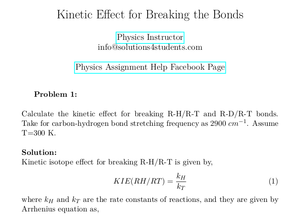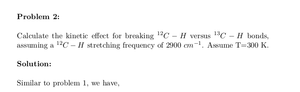Solved Problems in Physics, Physical Chemistry, and Computer Simulation Projects for College and Graduate Students.# Kinetic Effect for Breaking the Bonds

Regular price
\$6.30
Sale price
\$6.30
Tax included.

Problem 1:
Calculate the kinetic effect for breaking R-H/R-T and R-D/R-T bonds.
Take for carbon-hydrogen bond stretching frequency as 2900 cm−1. Assume T=300 K.

Problem 2:
Calculate the kinetic effect for breaking 12C − H versus 13C − H bonds, assuming a 12C − H stretching frequency of 2900 cm−1 . Assume T=300 K.

By purchasing this product, you will get the step by step solution of the above problems in pdf format and the corresponding latex file where you can edit the solution. I am also available to help  you with any possible question you may have.